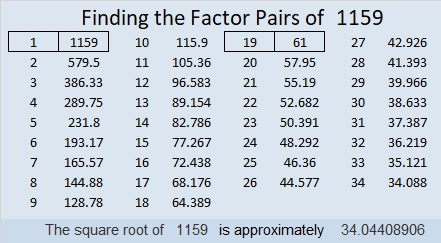# 1159 Mystery Level

This mysterious multiplication table does not have its factors in the usual places. Can you use logic to figure out where the factors from 1 to 10 go so that the given clues belong where they are?Print the puzzles or type the solution in this excel file: 10-factors-1148-1160

What can I tell you about the number 1159?

• 1159 is a composite number.
• Prime factorization: 1159 = 19 × 61
• The exponents in the prime factorization are 1 and 1. Adding one to each and multiplying we get (1 + 1)(1 + 1) = 2 × 2 = 4. Therefore 1159 has exactly 4 factors.
• Factors of 1159: 1, 19, 61, 1159
• Factor pairs: 1159 = 1 × 1159 or 19 × 61
• 1159 has no square factors that allow its square root to be simplified. √1159 ≈ 34.044091159 is the sum of the twenty-five prime numbers from 3 to 101.

1159 is the hypotenuse of a Pythagorean triple:
209-1140-1159 which is 19 times (11-60-61)

This site uses Akismet to reduce spam. Learn how your comment data is processed.